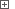# Obtaining Selected Rows in Syncfusion GridControlWhen testing your application with Syncfusion GridControl, you may need to select multiple rows in a grid. Then, you may need to determine which rows are selected in the grid in order to process them a certain way. You can obtain the selected rows using the following statement:

GridObj.Selections.GetSelectedRows( true, false )

where GridObj is the GridControl object. The first parameter of the `GetSelectedRows` method (True) specifies that only whole selected rows should be returned by the method. The second parameter (False) specifies that the returned ranges collection should not include the current cell.

This method returns the `GridRangeInfoList` object representing the collection of selected row ranges. To get the number of ranges in the collection, use the `Count` property. The `Item` property lets you access `GridRangeInfo` objects corresponding to individual ranges by their zero-based indexes within the collection. To determine which grid rows belong to a particular range object, you can use the `Top` and `Bottom` properties that hold indexes of the topmost and the lower-most rows of the given range.

 Note: In order for TestComplete to access the mentioned properties and methods of the GridControl object and its helper objects, the .NET Application Support plugin must be installed and enabled.

After you have determined the indexes of the selected grid rows, you can process the rows in a specific way. For example, you can iterate through them and obtain their cell values.

Below is an example that illustrates how you can process the selected rows in the GridControl.

###### ExampleView description

JavaScript, JScript

function Main ()
{
var p, Grid, RowIndexes, i;

// Obtain the application process and the grid object
p = Sys.Process("GridControlSort");
Grid = p.WinFormsObject("Form1").WinFormsObject("gridControl1");

// Get selected rows' indexes
RowIndexes = GetSelectedRowIndexes (Grid);

// Iterate through the selected rows
for (i=0; i<RowIndexes.length; i++)
Log.Message (GetCellText(Grid, RowIndexes[i], 1));
}

function GetSelectedRowIndexes (Grid)
{
var Ranges, Rng, Indexes, i, Idx;

Indexes = new Array ();
// Get the collection of selected row ranges
Ranges = Grid.Selections.GetSelectedRows(true, false);
// Iterate through the ranges collection
for (i=0; i<Ranges.Count; i++)
{
Rng = Ranges.Item(i);
// Get indexes of rows that belong to the current range
for (Idx=Rng.Top; Idx<=Rng.Bottom; Idx++)
Indexes.push(Idx);
}

return Indexes;
}

function GetCellText (Grid, RowIndex, ColIndex)
{
return Grid.Item(RowIndex, ColIndex).FormattedText.OleValue;
}

Python

``````def Main ():

# Obtain the grid object
p = Sys.Process("MultipleRecordSelection")
Grid = p.WinFormsObject("Form1").WinFormsObject("gridGroupingControl1")

# Select a range of rows
SelectRange (Grid, 7, 21)

# Obtain the selected rows
RowIndexes = GetSelectedRowIndexes (Grid, None)
# Iterate through the selected rows
for i in range(0, RowIndexes.length-1):
Log.Message ("CompanyName: " + Grid.wValue(RowIndexes[i], "CompanyName").ToString().OleValue)

# Selects the specified range of rows
def SelectRange (View, StartRowIndex, EndRowIndex):
# Select the first row of the range
View.ClickRowIndicator (StartRowIndex)
# Extend the selection
View.ClickRowIndicator (EndRowIndex, skShift)

# Returns an array of selected rows' indexes
def GetSelectedRowIndexes (Grid, TableOrGroup):

# Get the selected records collection
if (TableOrGroup == None):
TableOrGroup = Grid.Table
SelectedRecords = Grid.Table.SelectedRecords
else:
SelectedRecords = Grid.GetTable(TableOrGroup.ParentTableDescriptor.Name).SelectedRecords

# Create an array and fill it with rows' indexes
arr = list(SelectedRecords.Count)
for i in range(0, SelectedRecords.Count-1):
arr[i] = TableOrGroup.Records.IndexOf( SelectedRecords.Item[i].Record )

return arr``````

VBScript

Sub Main
Dim p, Grid, RowIndexes, i

' Obtain the application process and the grid object
Set p = Sys.Process("GridControlSort")
Set Grid = p.WinFormsObject("Form1").WinFormsObject("gridControl1")

' Get selected rows' indexes
RowIndexes = GetSelectedRowIndexes (Grid)

' Iterate through the selected rows
For i=0 To UBound(RowIndexes)
Call Log.Message (GetCellText(Grid, RowIndexes(i), 1))
Next
End Sub

Function GetSelectedRowIndexes (Grid)
Dim Ranges, Rng, Indexes, i, j, Idx

Indexes = Array ()

' Get the collection of selected row ranges
Set Ranges = Grid.Selections.GetSelectedRows(True, False)

' Iterate through the ranges collection
j = 0 ' Counter of Indexes elements
For i=0 To Ranges.Count-1
Set Rng = Ranges.Item(i)
' Increase the array size
Redim Preserve Indexes (UBound(Indexes) + Rng.Height)
' Get indexes of rows that belong to the current range
For Idx=Rng.Top To Rng.Bottom
Indexes(j) = Idx
j = j + 1
Next
Next

GetSelectedRowIndexes = Indexes
End Function

Function GetCellText (Grid, RowIndex, ColIndex)
GetCellText = Grid.Item(RowIndex, ColIndex).FormattedText.OleValue
End Function

DelphiScript

function GetSelectedRowIndexes (Grid); forward;
function GetCellText (Grid, RowIndex, ColIndex); forward;

procedure Main;
var p, Grid, RowIndexes, i : OleVariant;
begin
// Obtain the application process and the grid object
p := Sys.Process('GridControlSort');
Grid := p.WinFormsObject('Form1').WinFormsObject('gridControl1');

// Get selected rows' indexes
RowIndexes := GetSelectedRowIndexes (Grid);

// Iterate through the selected rows
for i:=0 to VarArrayHighBound(RowIndexes, 1) do
Log.Message (GetCellText(Grid, RowIndexes[i], 1));
end;

function GetSelectedRowIndexes (Grid);
var Ranges, Rng, Indexes, i, j, Idx : OleVariant;
begin
Indexes := CreateVariantArray(-1,-1);
// Get the collection of selected row ranges
Ranges := Grid.Selections.GetSelectedRows(true, false);
// Iterate through the ranges collection
j := 0; // Counter of Indexes elements
for i:=0 To Ranges.Count-1 do
begin
Rng := Ranges.Item[i];
// Increase the array size
VarArrayRedim (Indexes, VarArrayHighBound(Indexes, 1) + Rng.Height);
// Get indexes of rows that belong to the current range
for Idx:=Rng.Top to Rng.Bottom do
begin
Indexes[j] := Idx;
j := j + 1;
end;
end;

Result := Indexes;
end;

function GetCellText (Grid, RowIndex, ColIndex);
begin
Result := Grid.Item[RowIndex, ColIndex].FormattedText.OleValue;
end;

C++Script, C#Script

function Main ()
{
var p, Grid, RowIndexes, i;

// Obtain the application process and the grid object
p = Sys["Process"]("GridControlSort");
Grid = p["WinFormsObject"]("Form1")["WinFormsObject"]("gridControl1");

// Get selected rows' indexes
RowIndexes = GetSelectedRowIndexes (Grid);

// Iterate through the selected rows
for (i=0; i<RowIndexes.length; i++)
Log["Message"](GetCellText(Grid, RowIndexes[i], 1));
}

function GetSelectedRowIndexes (Grid)
{
var Ranges, Rng, Indexes, i, Idx;

Indexes = new Array ();
// Get the collection of selected row ranges
Ranges = Grid["Selections"]["GetSelectedRows"](true, false);
// Iterate through the ranges collection
for (i=0; i<Ranges["Count"]; i++)
{
Rng = Ranges["Item"](i);
// Get indexes of rows that belong to the current range
for (Idx=Rng["Top"]; Idx<=Rng["Bottom"]; Idx++)
Indexes["push"](Idx);
}

return Indexes;
}

function GetCellText (Grid, RowIndex, ColIndex)
{
return Grid["Item"](RowIndex, ColIndex)["FormattedText"]["OleValue"];
}